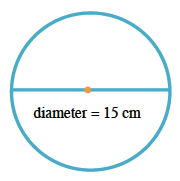Home > ACC7 > Chapter cc33 > Lesson cc33.2.2 > Problem3-86

3-86.

Find the area and circumference of a circle with a diameter of $15$ cm. If you need help remembering how to do this, read the Math Notes box in this lesson. Homework Help ✎

Remember the the area of a circle is $\pi r^2$ and the circumference of a circle is $2\pi r$.

The radius of a circle is half the diameter.

radius $=7.5$ cm

Area $=\pi·(7.5\text{ cm})^2=\pi·(7.5\text{ cm})(7.5\text{ cm})=56.25\pi \text{ cm}^2\approx176.7\text{ cm}^2$

Circumference $=2\pi·(7.5\text{ cm})=15\pi \text{ cm}\approx47.1\text{ cm}$